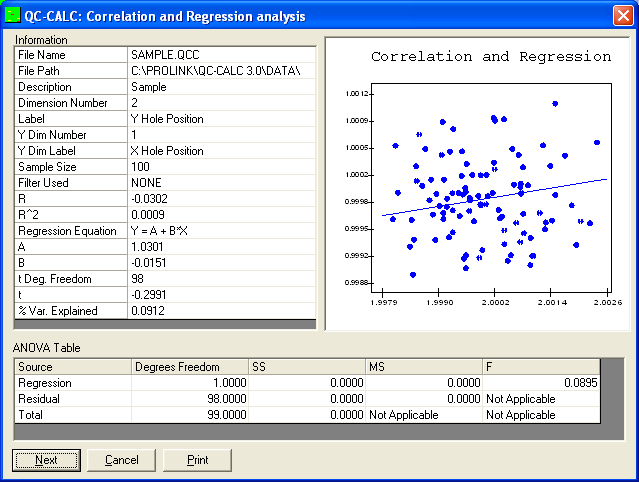Correlation & Regression
Correlation & Regression

The Correlation and Regression Analysis is used to show the relationship of one characteristic to another. If a dependent relationship exists the regression plot will indicate strong correlation. If the two characteristics analyzed have little or no relationship, the regression plot will indicate little correlation. The amount of correlation is visually determined by observing how the data points behave. A tightly grouped pattern over the range of the plot generally indicates a stronger relationship than a loosely grouped pattern.

Uses of Regression Analysis
• To determine the relationship between two process variables. If a cause and effect is present the regression analysis will indicate strong correlation.
• Identification of the key variable or variables which contribute to variation in other characteristics. Isolating the causes of variation provides knowledge of which characteristics should be continuously monitored for process control.